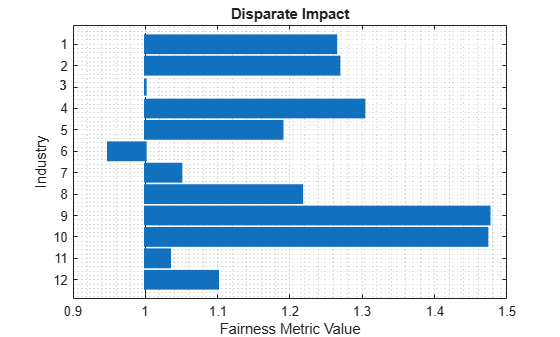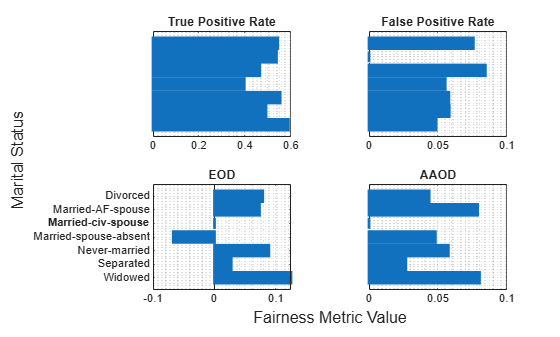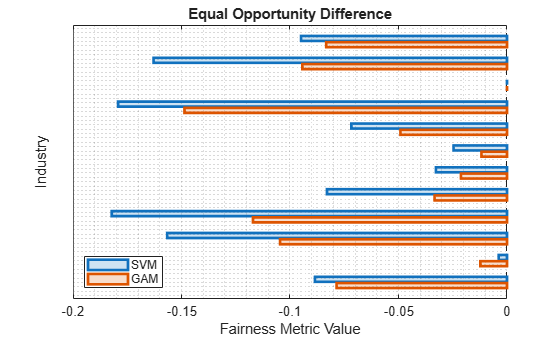# plot

Plot bar graph of fairness metric

Since R2022b

## Syntax

``plot(evaluator,metric)``
``plot(evaluator,metric,Name=Value)``
``b = plot(___)``

## Description

example

````plot(evaluator,metric)` creates a bar graph of the specified metric (`metric`), stored in either the `BiasMetrics` or `GroupMetrics` property of the `fairnessMetrics` object (`evaluator`). By default, the function creates a graph for the first attribute stored in the `SensitiveAttributeNames` property of `evaluator`.If you specified predicted class labels for multiple models when you created `evaluator`, then the graph includes bars of different colors, where the color indicates the model.```

example

````plot(evaluator,metric,Name=Value)` specifies additional options using one or more name-value arguments. For example, you can specify the sensitive attribute to plot by using the `SensitiveAttributeName` name-value argument.```

example

````b = plot(___)` returns a `Bar` object or an array of `Bar` objects. Use `b` to query or modify Bar Properties after displaying the bar graph.```

## Examples

collapse all

Compute fairness metrics for true labels with respect to sensitive attributes by creating a `fairnessMetrics` object. Then, plot a bar graph of a specified metric by using the `plot` function.

Read the sample file `CreditRating_Historical.dat` into a table. The predictor data consists of financial ratios and industry sector information for a list of corporate customers. The response variable consists of credit ratings assigned by a rating agency.

`creditrating = readtable("CreditRating_Historical.dat");`

Because each value in the `ID` variable is a unique customer ID—that is, `length(unique(creditrating.ID))` is equal to the number of observations in `creditrating`—the `ID` variable is a poor predictor. Remove the `ID` variable from the table, and convert the `Industry` variable to a `categorical` variable.

```creditrating.ID = []; creditrating.Industry = categorical(creditrating.Industry);```

In the `Rating` response variable, combine the `AAA`, `AA`, `A`, and `BBB` ratings into a category of "good" ratings, and the `BB`, `B`, and `CCC` ratings into a category of "poor" ratings.

```Rating = categorical(creditrating.Rating); Rating = mergecats(Rating,["AAA","AA","A","BBB"],"good"); Rating = mergecats(Rating,["BB","B","CCC"],"poor"); creditrating.Rating = Rating;```

Compute fairness metrics with respect to the sensitive attribute `Industry` for the labels in the `Rating` variable.

```evaluator = fairnessMetrics(creditrating,"Rating", ... SensitiveAttributeNames="Industry");```

`fairnessMetrics` computes metrics for all supported bias and group metrics. Display the names of the metrics stored in the `BiasMetrics` and `GroupMetrics` properties.

`evaluator.BiasMetrics.Properties.VariableNames(3:end)'`
```ans = 2x1 cell {'StatisticalParityDifference'} {'DisparateImpact' } ```
`evaluator.GroupMetrics.Properties.VariableNames(3:end)'`
```ans = 2x1 cell {'GroupCount' } {'GroupSizeRatio'} ```

Create a bar graph of the disparate impact values.

`plot(evaluator,"DisparateImpact")`Compute fairness metrics for predicted labels with respect to sensitive attributes by creating a `fairnessMetrics` object. Then, plot a bar graph of a specified metric and sensitive attribute by using the `plot` function.

Load the sample data `census1994`, which contains the training data `adultdata` and the test data `adulttest`. The data sets consist of demographic information from the US Census Bureau that can be used to predict whether an individual makes over \$50,000 per year. Preview the first few rows of the training data set.

```load census1994 head(adultdata)```
``` age workClass fnlwgt education education_num marital_status occupation relationship race sex capital_gain capital_loss hours_per_week native_country salary ___ ________________ __________ _________ _____________ _____________________ _________________ _____________ _____ ______ ____________ ____________ ______________ ______________ ______ 39 State-gov 77516 Bachelors 13 Never-married Adm-clerical Not-in-family White Male 2174 0 40 United-States <=50K 50 Self-emp-not-inc 83311 Bachelors 13 Married-civ-spouse Exec-managerial Husband White Male 0 0 13 United-States <=50K 38 Private 2.1565e+05 HS-grad 9 Divorced Handlers-cleaners Not-in-family White Male 0 0 40 United-States <=50K 53 Private 2.3472e+05 11th 7 Married-civ-spouse Handlers-cleaners Husband Black Male 0 0 40 United-States <=50K 28 Private 3.3841e+05 Bachelors 13 Married-civ-spouse Prof-specialty Wife Black Female 0 0 40 Cuba <=50K 37 Private 2.8458e+05 Masters 14 Married-civ-spouse Exec-managerial Wife White Female 0 0 40 United-States <=50K 49 Private 1.6019e+05 9th 5 Married-spouse-absent Other-service Not-in-family Black Female 0 0 16 Jamaica <=50K 52 Self-emp-not-inc 2.0964e+05 HS-grad 9 Married-civ-spouse Exec-managerial Husband White Male 0 0 45 United-States >50K ```

Each row contains the demographic information for one adult. The information includes sensitive attributes, such as `age`, `marital_status`, `relationship`, `race`, and `sex`. The third column `flnwgt` contains observation weights, and the last column `salary` shows whether a person has a salary less than or equal to \$50,000 per year (`<=50K`) or greater than \$50,000 per year (`>50K`).

Train a classification tree using the training data set `adultdata`. Specify the response variable, predictor variables, and observation weights by using the variable names in the `adultdata` table.

```predictorNames = ["capital_gain","capital_loss","education", ... "education_num","hours_per_week","occupation","workClass"]; Mdl = fitctree(adultdata,"salary", ... PredictorNames=predictorNames,Weights="fnlwgt");```

Predict the test sample labels by using the trained tree `Mdl`.

`adulttest.predictions = predict(Mdl,adulttest);`

This example evaluates the fairness of the predicted labels with respect to age and marital status. Group the `age` variable into four bins.

```ageGroups = ["Age<30","30<=Age<45","45<=Age<60","Age>=60"]; adulttest.age_group = discretize(adulttest.age, ... [min(adulttest.age) 30 45 60 max(adulttest.age)], ... categorical=ageGroups);```

Compute fairness metrics for the predictions with respect to the `age_group` and `marital_status` variables by using `fairnessMetrics`.

```MdlEvaluator = fairnessMetrics(adulttest,"salary", ... SensitiveAttributeNames=["age_group","marital_status"], ... Predictions="predictions",Weights="fnlwgt")```
```MdlEvaluator = fairnessMetrics with properties: SensitiveAttributeNames: {'age_group' 'marital_status'} ReferenceGroup: {'30<=Age<45' 'Married-civ-spouse'} ResponseName: 'salary' PositiveClass: >50K BiasMetrics: [11x7 table] GroupMetrics: [11x20 table] ModelNames: 'predictions' Properties, Methods ```

Create bar graphs of the true positive rate (TPR), false positive rate (FPR), equal opportunity difference (EOD), and average absolute odds difference (AAOD) values for the sensitive attribute `marital_status`. The default value of the `SensitiveAttributeName` argument is the first element in the `SensitiveAttributeNames` property of the `fairnessMetrics` object. In this case, the first element is `age_group`. Specify `SensitiveAttributeName` as `marital_status`.

```t = tiledlayout(2,2); nexttile plot(MdlEvaluator,"tpr",SensitiveAttributeName="marital_status") xlabel("") ylabel("") nexttile plot(MdlEvaluator,"fpr",SensitiveAttributeName="marital_status") yticklabels("") xlabel("") ylabel("") nexttile plot(MdlEvaluator,"eod",SensitiveAttributeName="marital_status") xlabel("") ylabel("") title("EOD") nexttile plot(MdlEvaluator,"aaod",SensitiveAttributeName="marital_status") yticklabels("") xlabel("") ylabel("") title("AAOD") xlabel(t,"Fairness Metric Value") ylabel(t,"Marital Status")```Train two classification models, and compare the model predictions by using fairness metrics.

Read the sample file `CreditRating_Historical.dat` into a table. The predictor data consists of financial ratios and industry sector information for a list of corporate customers. The response variable consists of credit ratings assigned by a rating agency.

`creditrating = readtable("CreditRating_Historical.dat");`

Because each value in the `ID` variable is a unique customer ID—that is, `length(unique(creditrating.ID))` is equal to the number of observations in `creditrating`—the `ID` variable is a poor predictor. Remove the `ID` variable from the table, and convert the `Industry` variable to a `categorical` variable.

```creditrating.ID = []; creditrating.Industry = categorical(creditrating.Industry);```

In the `Rating` response variable, combine the `AAA`, `AA`, `A`, and `BBB` ratings into a category of "good" ratings, and the `BB`, `B`, and `CCC` ratings into a category of "poor" ratings.

```Rating = categorical(creditrating.Rating); Rating = mergecats(Rating,["AAA","AA","A","BBB"],"good"); Rating = mergecats(Rating,["BB","B","CCC"],"poor"); creditrating.Rating = Rating;```

Train a support vector machine (SVM) model on the `creditrating` data. For better results, standardize the predictors before fitting the model. Use the trained model to predict labels and compute the misclassification rate for the training data set.

```predictorNames = ["WC_TA","RE_TA","EBIT_TA","MVE_BVTD","S_TA"]; SVMMdl = fitcsvm(creditrating,"Rating", ... PredictorNames=predictorNames,Standardize=true); SVMPredictions = resubPredict(SVMMdl); resubLoss(SVMMdl)```
```ans = 0.0872 ```

Train a generalized additive model (GAM).

```GAMMdl = fitcgam(creditrating,"Rating", ... PredictorNames=predictorNames); GAMPredictions = resubPredict(GAMMdl); resubLoss(GAMMdl)```
```ans = 0.0542 ```

`GAMMdl` achieves better accuracy on the training data set.

Compute fairness metrics with respect to the sensitive attribute `Industry` by using the model predictions for both models.

```predictions = [SVMPredictions,GAMPredictions]; evaluator = fairnessMetrics(creditrating,"Rating", ... SensitiveAttributeNames="Industry",Predictions=predictions, ... ModelNames=["SVM","GAM"]);```

Display the bias metrics by using the `report` function.

`report(evaluator)`
```ans=48×5 table Metrics SensitiveAttributeNames Groups SVM GAM ___________________________ _______________________ ______ _________ __________ StatisticalParityDifference Industry 1 -0.028441 0.0058208 StatisticalParityDifference Industry 2 -0.04014 0.0063339 StatisticalParityDifference Industry 3 0 0 StatisticalParityDifference Industry 4 -0.04905 -0.0043007 StatisticalParityDifference Industry 5 -0.015615 0.0041607 StatisticalParityDifference Industry 6 -0.03818 -0.024515 StatisticalParityDifference Industry 7 -0.01514 0.007326 StatisticalParityDifference Industry 8 0.0078632 0.036581 StatisticalParityDifference Industry 9 -0.013863 0.042266 StatisticalParityDifference Industry 10 0.0090218 0.050095 StatisticalParityDifference Industry 11 -0.004188 0.001453 StatisticalParityDifference Industry 12 -0.041572 -0.028589 DisparateImpact Industry 1 0.92261 1.017 DisparateImpact Industry 2 0.89078 1.0185 DisparateImpact Industry 3 1 1 DisparateImpact Industry 4 0.86654 0.98742 ⋮ ```

Among the bias metrics, compare the equal opportunity difference (EOD) values. Create a bar graph of the EOD values by using the `plot` function.

```b = plot(evaluator,"eod"); b(1).FaceAlpha = 0.2; b(2).FaceAlpha = 0.2; legend(Location="southwest")```To better understand the distributions of EOD values, plot the values using box plots.

```boxchart(evaluator.BiasMetrics.EqualOpportunityDifference, ... GroupByColor=evaluator.BiasMetrics.ModelNames) ax = gca; ax.XTick = []; ylabel("Equal Opportunity Difference") legend```The EOD values for GAM are closer to 0 compared to the values for SVM.

## Input Arguments

collapse all

Object containing fairness metrics, specified as a `fairnessMetrics` object.

Fairness metric to plot, specified as a bias or group metric stored in either the `BiasMetrics` or `GroupMetrics` property of the `fairnessMetrics` object (`evaluator`). The properties in `evaluator` use full names for the table variable names. However, you can use either the full name or short name given in the following tables to specify the `metric` argument.

• Bias metrics

Metric NameDescriptionEvaluation Type
`"StatisticalParityDifference"` or `"spd"`Statistical parity difference (SPD)Data-level or model-level evaluation
`"DisparateImpact"` or `"di"`Disparate impact (DI)Data-level or model-level evaluation
`"EqualOpportunityDifference"` or `"eod"`Equal opportunity difference (EOD)Model-level evaluation
`"AverageAbsoluteOddsDifference"` or `"aaod"`Average absolute odds difference (AAOD)Model-level evaluation

For definitions of the bias metrics, see Bias Metrics.

• Group metrics

Metric NameDescriptionEvaluation Type
`"GroupCount"`Group count, or number of samples in the groupData-level or model-level evaluation
`"GroupSizeRatio"`Group count divided by the total number of samplesData-level or model-level evaluation
`"TruePositives"` or `"tp"`Number of true positives (TP)Model-level evaluation
`"TrueNegatives"` or `"tn"`Number of true negatives (TN)Model-level evaluation
`"FalsePositives"` or `"fp"`Number of false positives (FP)Model-level evaluation
`"FalseNegatives"` or `"fn"`Number of false negatives (FN)Model-level evaluation
`"TruePositiveRate"` or `"tpr"`True positive rate (TPR), also known as recall or sensitivity, `TP/(TP+FN)`Model-level evaluation
`"TrueNegativeRate"`, `"tnr"`, or `"spec"`True negative rate (TNR), or specificity, `TN/(TN+FP)`Model-level evaluation
`"FalsePositiveRate"` or `"fpr"`False positive rate (FPR), also known as fallout or 1-specificity, `FP/(TN+FP)`Model-level evaluation
`"FalseNegativeRate"`, `"fnr"`, or `"miss"`False negative rate (FNR), or miss rate, `FN/(TP+FN)`Model-level evaluation
`"FalseDiscoveryRate"` or `"fdr"`False discovery rate (FDR), `FP/(TP+FP)`Model-level evaluation
`"FalseOmissionRate"` or `"for"`False omission rate (FOR), `FN/(TN+FN)`Model-level evaluation
`"PositivePredictiveValue"`, `"ppv"`, or `"prec"`Positive predictive value (PPV), or precision, `TP/(TP+FP)`Model-level evaluation
`"NegativePredictiveValue"` or `"npv"`Negative predictive value (NPV), `TN/(TN+FN)`Model-level evaluation
`"RateOfPositivePredictions"` or `"rpp"`Rate of positive predictions (RPP), `(TP+FP)/(TP+FN+FP+TN)`Model-level evaluation
`"RateOfNegativePredictions"` or `"rnp"`Rate of negative predictions (RNP), `(TN+FN)/(TP+FN+FP+TN)`Model-level evaluation
`"Accuracy"` or `"accu"`Accuracy, `(TP+TN)/(TP+FN+FP+TN)`Model-level evaluation

A `fairnessMetrics` object stores bias and group metrics in the `BiasMetrics` and `GroupMetrics` properties, respectively. The supported metrics depend on whether you specify predicted labels by using the `Predictions` argument when you create the `fairnessMetrics` object.

• Data-level evaluation — If you specify true labels and do not specify predicted labels, the `BiasMetrics` property contains only `StatisticalParityDifference` and `DisparateImpact`, and the `GroupMetrics` property contains only `GroupCount` and `GroupSizeRatio`.

• Model-level evaluation — If you specify both true labels and predicted labels, `BiasMetrics` and `GroupMetrics` contain all metrics listed in the tables.

Data Types: `char` | `string`

### Name-Value Arguments

Specify optional pairs of arguments as `Name1=Value1,...,NameN=ValueN`, where `Name` is the argument name and `Value` is the corresponding value. Name-value arguments must appear after other arguments, but the order of the pairs does not matter.

Example: `SensitiveAttributeName="Age",ModelNames="Tree"` specifies to plot fairness metric values for the `Age` sensitive attribute, computed using the `Tree` model predicted labels.

Name of the sensitive attribute to plot, specified as a character vector or string scalar. The sensitive attribute name must be a name in the `SensitiveAttributeNames` property of `evaluator`.

Example: `SensitiveAttributeName="race"`

Data Types: `char` | `string`

Since R2023a

Names of the models to plot, specified as `"all"`, a character vector, a string array, or a cell array of character vectors. The `ModelNames` value must contain names in the `ModelNames` property of `evaluator`. Using the `"all"` value is equivalent to specifying `evaluator.ModelNames`.

Example: `ModelNames="Tree"`

Example: `ModelNames=["SVM","Neural Network"]`

Data Types: `char` | `string` | `cell`

collapse all

### Bias Metrics

The `fairnessMetrics` object supports four bias metrics: statistical parity difference (SPD), disparate impact (DI), equal opportunity difference (EOD), and average absolute odds difference (AAOD). The object supports EOD and AAOD only for evaluating model predictions.

A `fairnessMetrics` object computes bias metrics for each group in each sensitive attribute with respect to the reference group of the attribute.

• Statistical parity (or demographic parity) difference (SPD)

The SPD value of the ith sensitive attribute (Si) for the group sij with respect to the reference group sir is defined by

`${\text{SPD}}_{ij}=P\left(Y=+|{S}_{i}={s}_{ij}\right)-P\left(Y=+|{S}_{i}={s}_{ir}\right).$`

The SPD value is the difference between the probability of being in the positive class when the sensitive attribute value is sij and the probability of being in the positive class when the sensitive attribute value is sir (reference group). This metric assumes that the two probabilities (statistical parities) are equal if the labels are unbiased with respect to the sensitive attribute.

If you specify the `Predictions` argument, the software computes SPD for the probabilities of the model predictions $\stackrel{^}{Y}$ instead of the true labels Y.

• Disparate impact (DI)

The DI value of the ith sensitive attribute (Si) for the group sij with respect to the reference group sir is defined by

`${\text{DI}}_{ij}=\frac{P\left(Y=+|{S}_{i}={s}_{ij}\right)}{P\left(Y=+|{S}_{i}={s}_{ir}\right)}.$`

The DI value is the ratio of the probability of being in the positive class when the sensitive attribute value is sij to the probability of being in the positive class when the sensitive attribute value is sir (reference group). This metric assumes that the two probabilities are equal if the labels are unbiased with respect to the sensitive attribute. In general, a DI value less than `0.8` or greater than `1.25` indicates bias with respect to the reference group .

If you specify the `Predictions` argument, the software computes DI for the probabilities of the model predictions $\stackrel{^}{Y}$ instead of the true labels Y.

• Equal opportunity difference (EOD)

The EOD value of the ith sensitive attribute (Si) for the group sij with respect to the reference group sir is defined by

`$\begin{array}{c}{\text{EOD}}_{ij}=\text{TPR}\left({S}_{i}={s}_{ij}\right)-\text{TPR}\left({S}_{i}={s}_{ir}\right)\\ =P\left(\stackrel{^}{Y}=+|Y=+,{S}_{i}={s}_{ij}\right)-P\left(\stackrel{^}{Y}=+|Y=+,{S}_{i}={s}_{ir}\right).\end{array}$`

The EOD value is the difference in the true positive rate (TPR) between the group sij and the reference group sir. This metric assumes that the two rates are equal if the predicted labels are unbiased with respect to the sensitive attribute.

• Average absolute odds difference (AAOD)

The AAOD value of the ith sensitive attribute (Si) for the group sij with respect to the reference group sir is defined by

`${\text{AAOD}}_{ij}=\frac{1}{2}\left(|\text{FPR}\left({S}_{i}={s}_{ij}\right)-\text{FPR}\left({S}_{i}={s}_{ir}\right)|+|\text{TPR}\left({S}_{i}={s}_{ij}\right)-\text{TPR}\left({S}_{i}={s}_{ir}\right)|\right).$`

The AAOD value represents the difference in the true positive rates (TPR) and false positive rates (FPR) between the group sij and the reference group sir. This metric assumes no difference in TPR and FPR if the predicted labels are unbiased with respect to the sensitive attribute.

 Mehrabi, Ninareh, et al. “A Survey on Bias and Fairness in Machine Learning.” ArXiv:1908.09635 [cs.LG], Sept. 2019. arXiv.org.

 Saleiro, Pedro, et al. “Aequitas: A Bias and Fairness Audit Toolkit.” ArXiv:1811.05577 [cs.LG], April 2019. arXiv.org.